# TouchMath Numbers 1 9 PrintableNumberMar 01, 2021By PrintableeNo Comment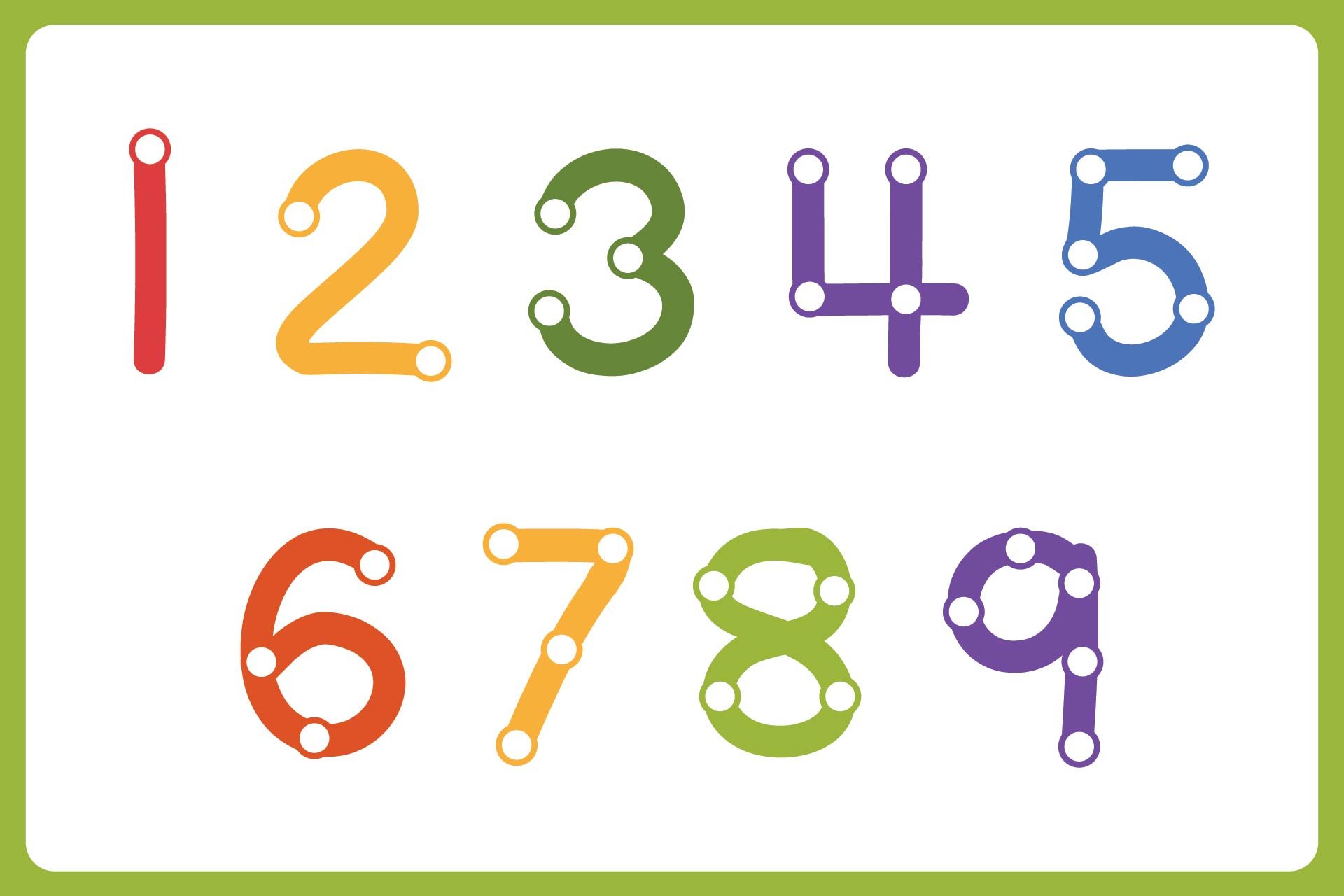### What is touch math strategy?

One of the recommended ways to make the introduction of mathematics lessons better is using touchmat numbers 1-9. The number 1-9 is chosen because it is all kinds of digits in the numbers. The touchmat itself refers to a multisensory method for mathematical lessons. This technique is programmed for pre kindergartens to the third graders. It is known to help the kids learn with different styles. It's because some kids have a problem receiving the auditory and visual approach. So, the tactile strategy is involved to cover all possible approaches to make them understand the concept of numbers and operations.

### How do you introduce touch in mat?

Inside the touchmat, there are numbers from 1 to 9 that are drawn or written in a single seamless line. Then, there is a dot put onto the number. The amount of the dot is the same as the number shown. If we write a number 1, then we only put one solid dot there. We only draw the point half the value of the number. Then, we pour the remaining points into a circle that surrounds the point. If we look at a solid point surrounded by a circle, that means we are multiplying the point twice. For example, when we make the number 8, we only add 4 solid points. Then, give circles to the four solid points. So, the calculated point is multiplied by two. Therefore, the number of points was calculated to be eight. For every solid dot we find, tap it and count it as one. Then, move our point fingers to another dot. If we reach the dot with a circle outside, count it as two. Then, tap twice. When we are tapping and moving the dots, make sure we also recite the current number of the dot that we point. This will be helpful to know the value of that number. So, when we do operations with this method, it can make the counting easier.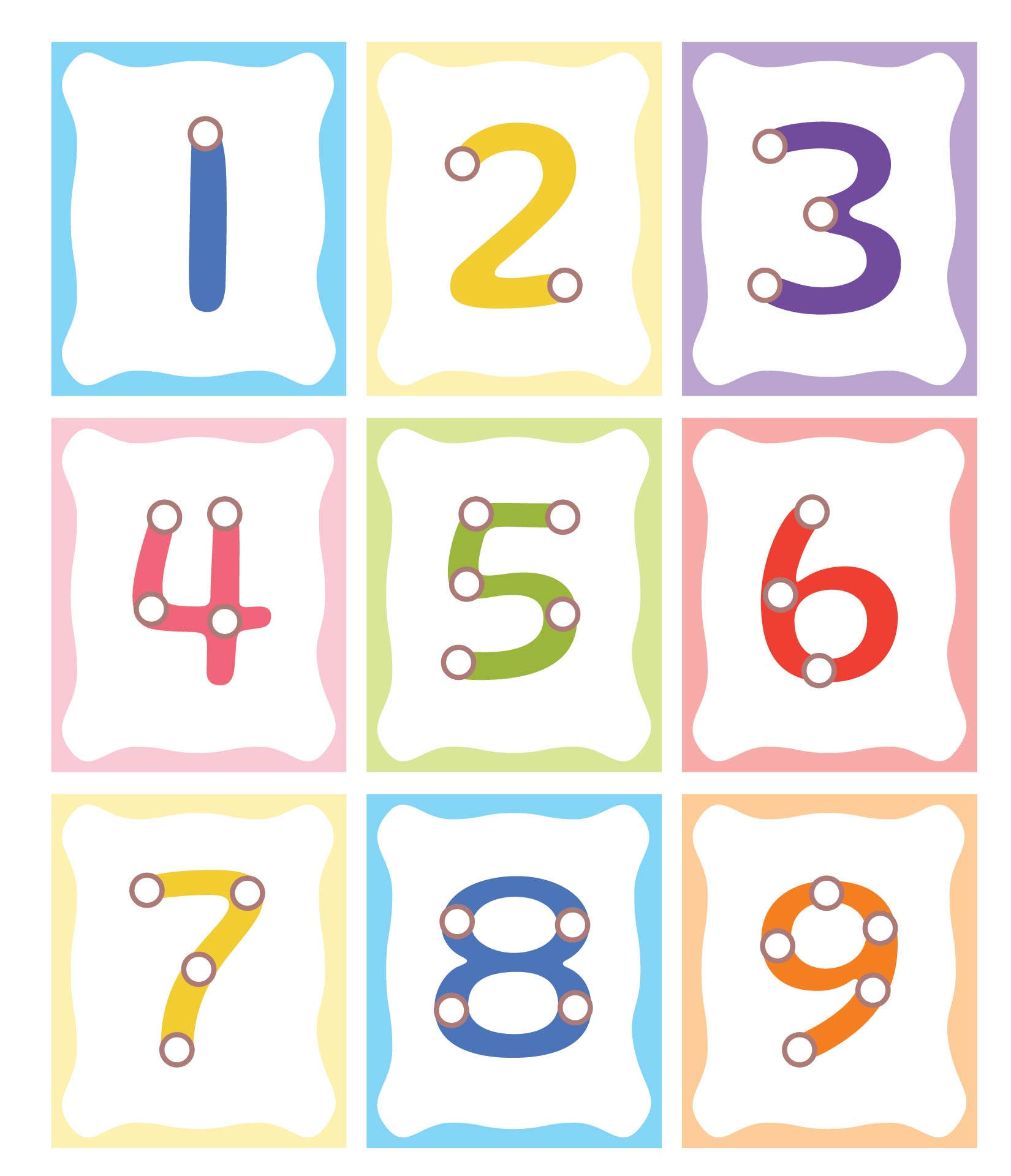We also have more printable number you may like:
Spanish Numbers 1 50 Printable
French Numbers 1 100 Printable
Large Printable Cut Out Numbers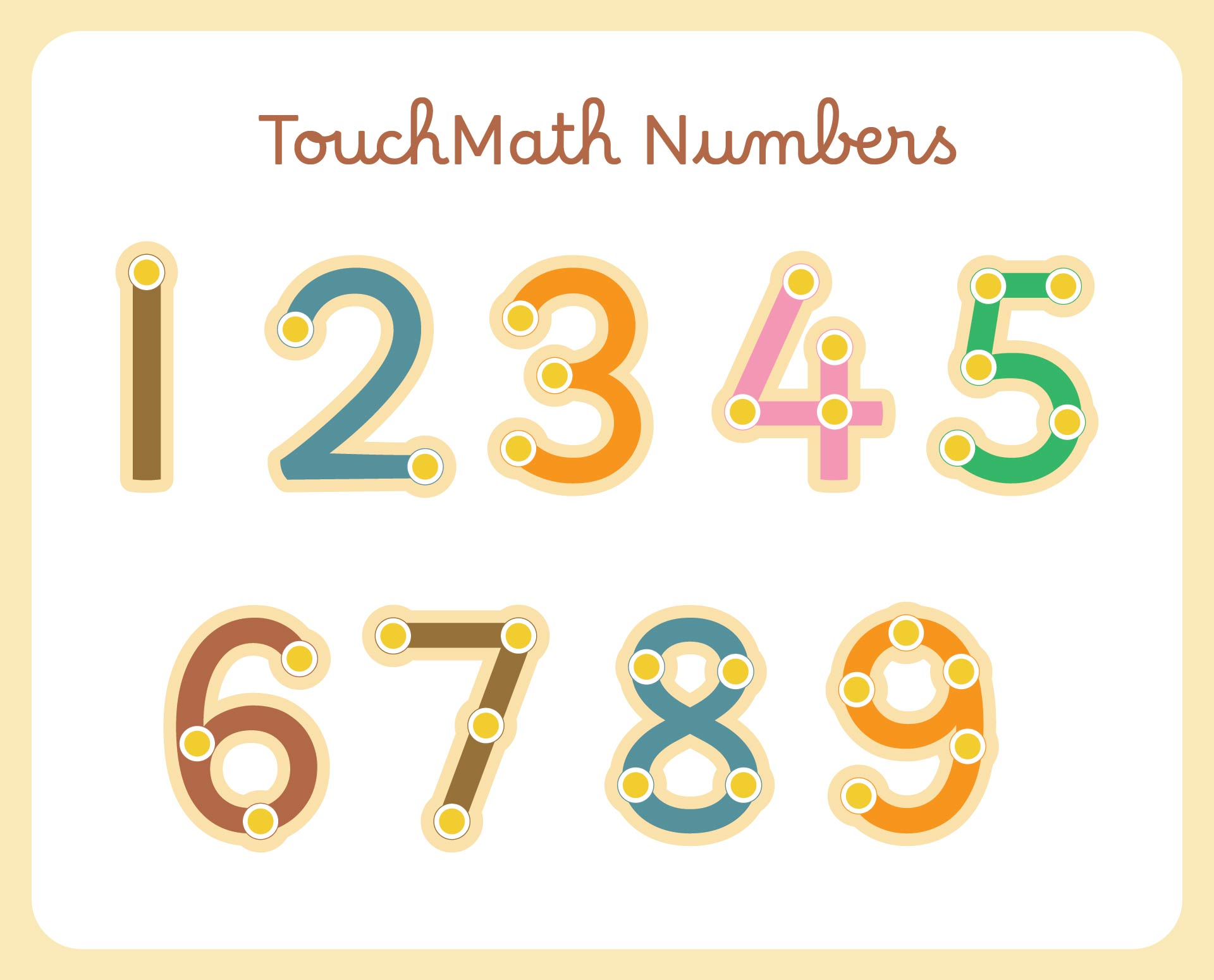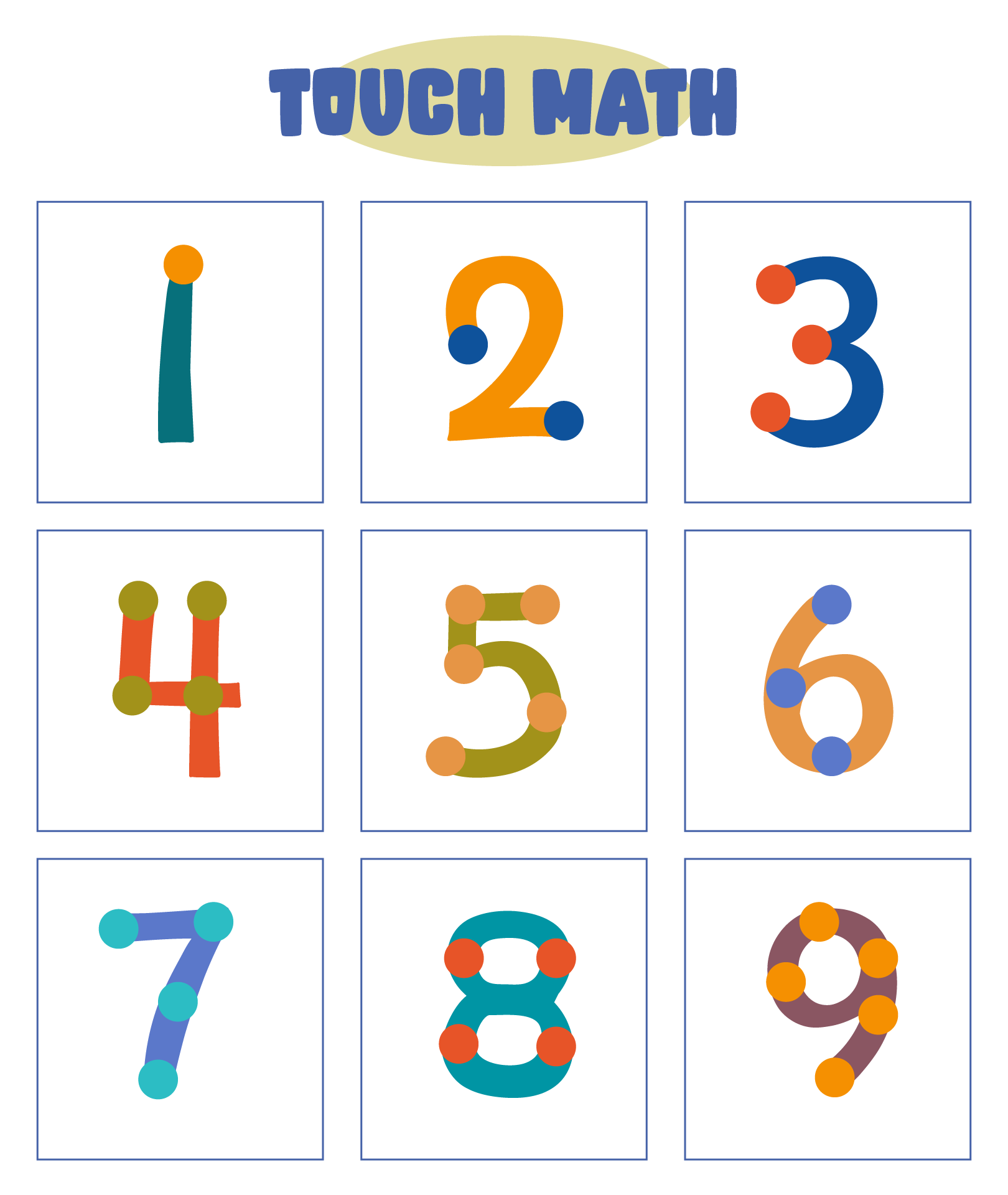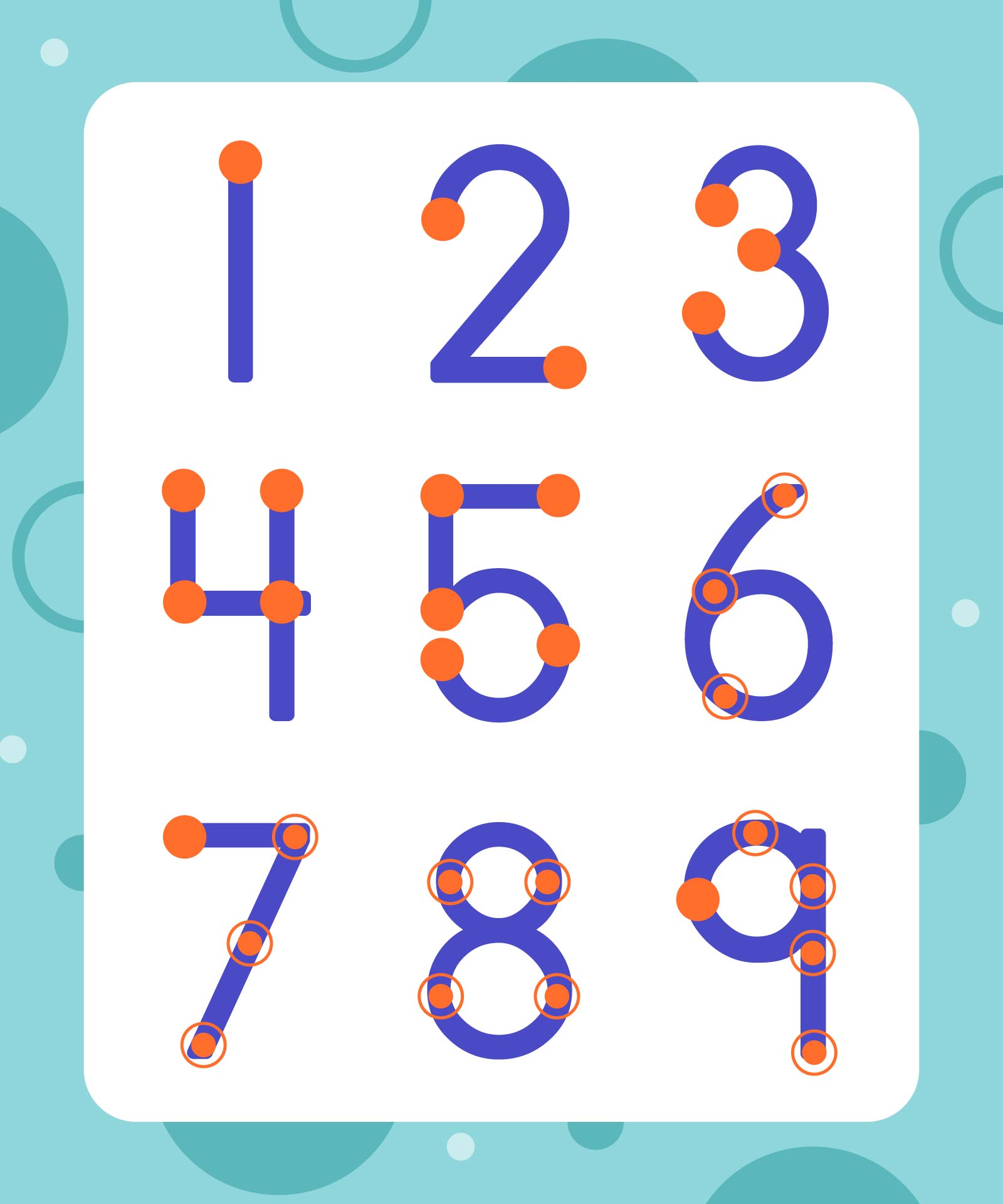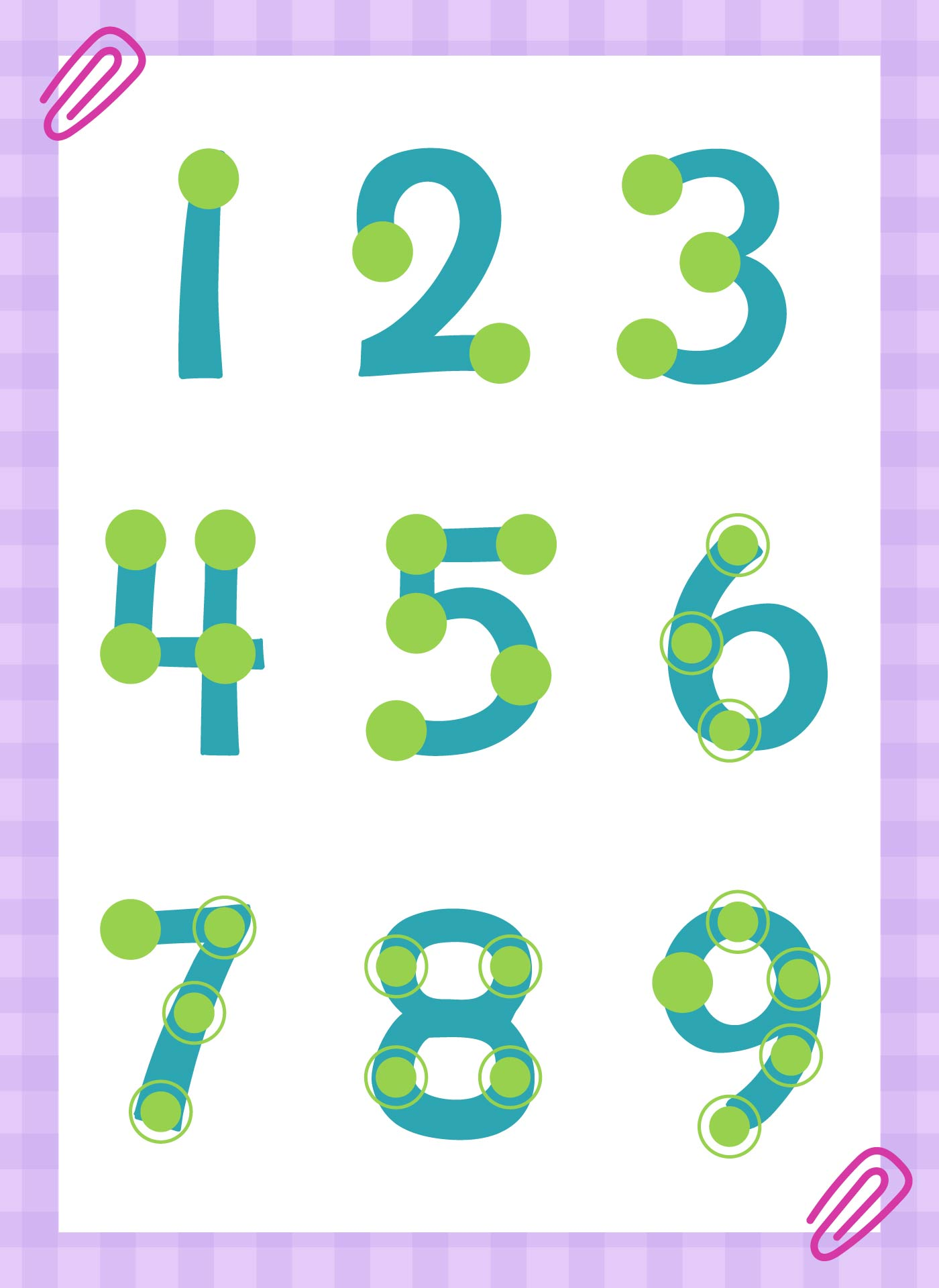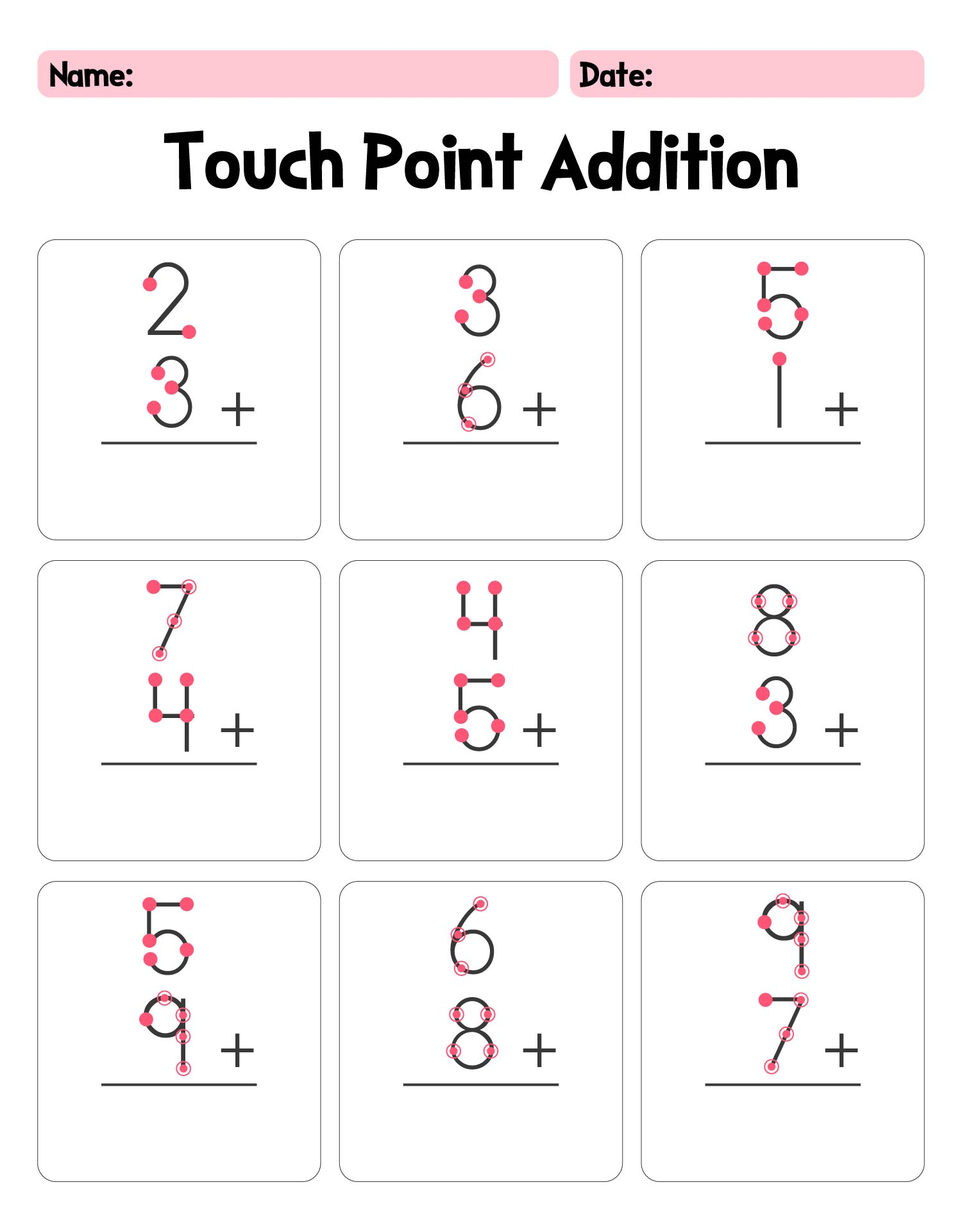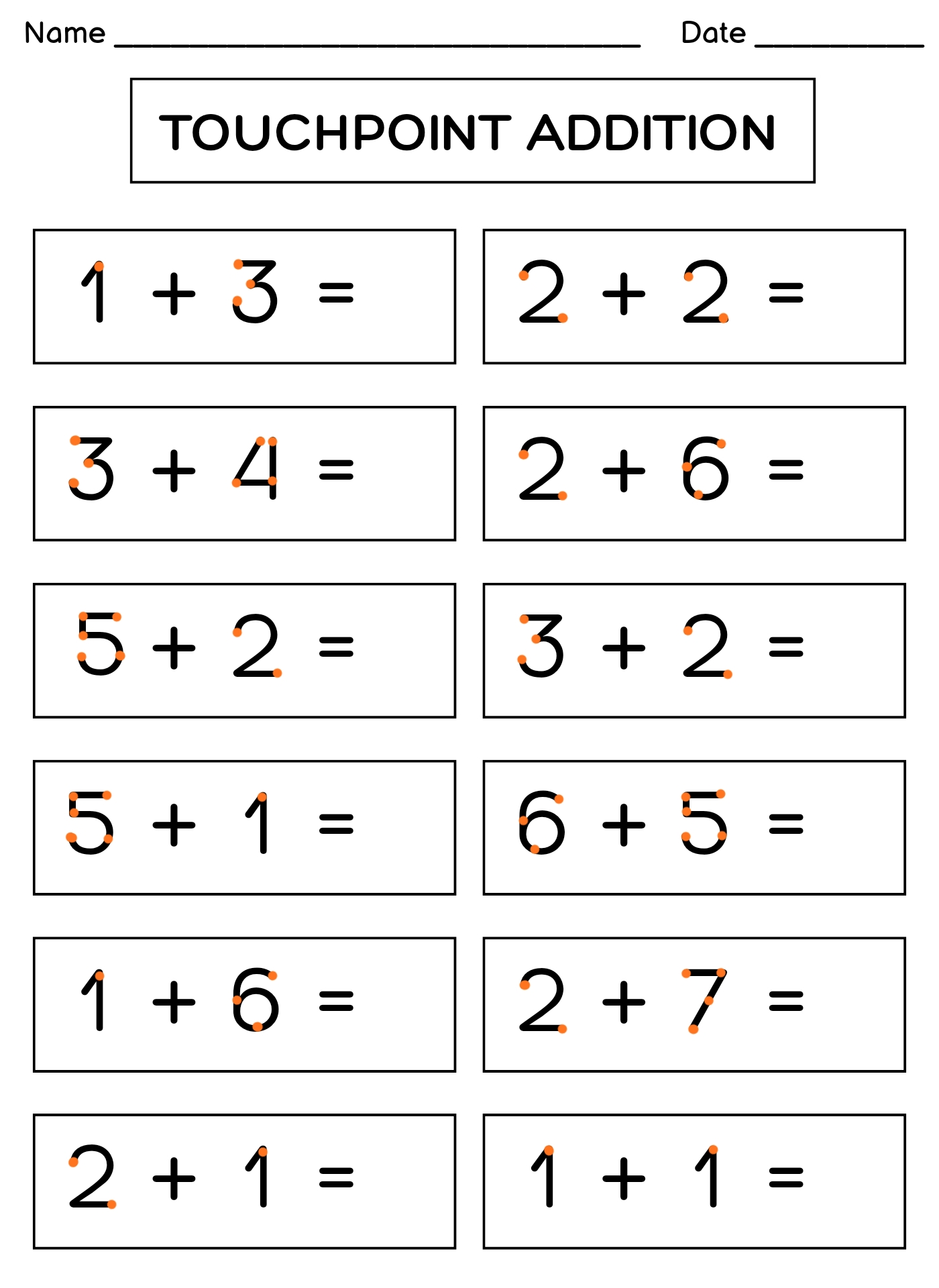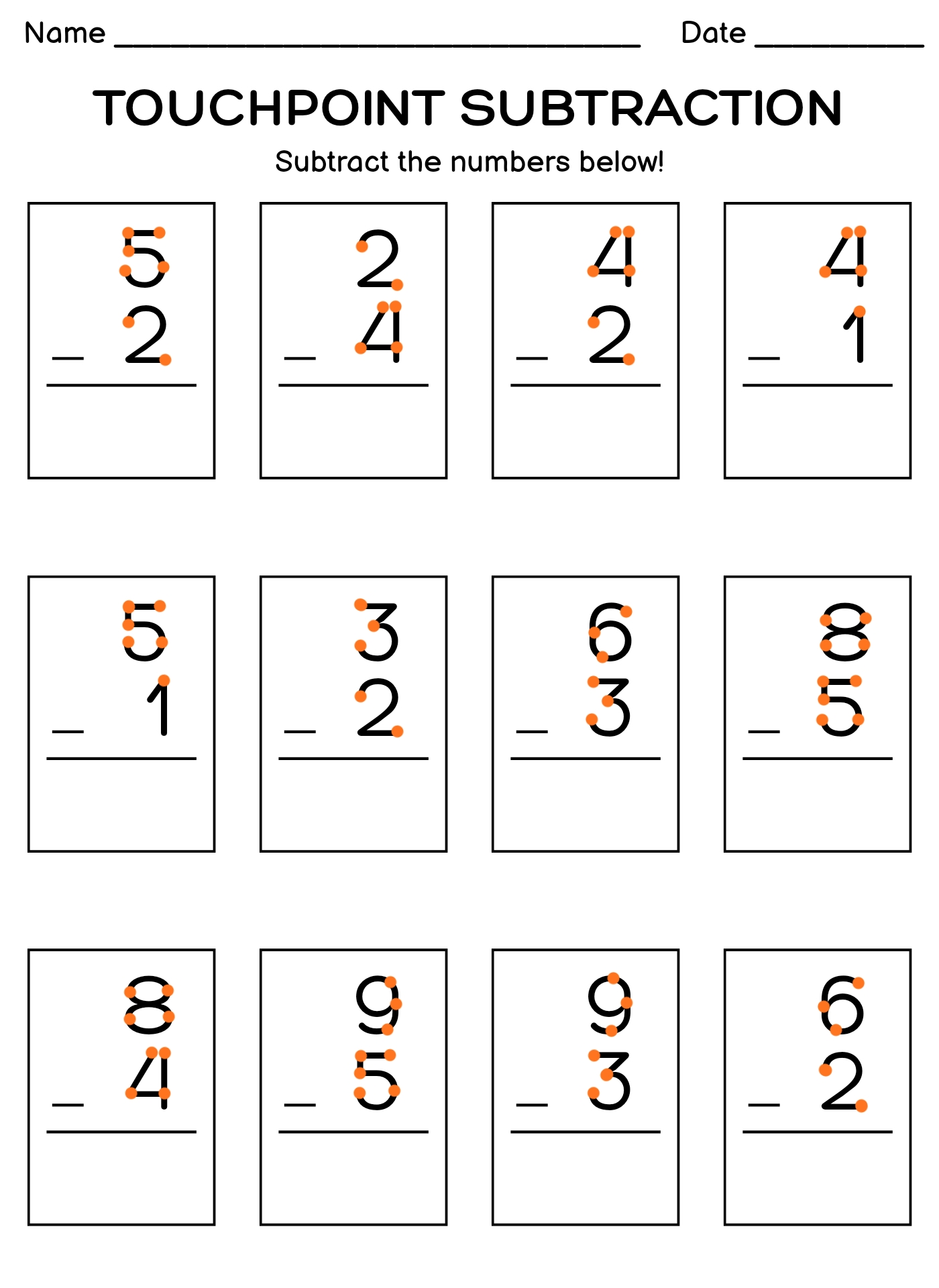### Is Touch Math evidence based?

Yes, touchmat has scientifically proven. There is a result of a research by the Department of Special Education, Counseling, and Student Affairs of Kansas State University that touchmat made the accurancy of mathematical operation with single digit addition significantly raised. Learning mathematical addition with touchmat can be considered as an effective method for students with most significant cognitive disabilities. In this study, touchmat was brought in a special education classroom that consists of three students with Autism Spectrum Disorder.